Yan, F., Xu, K., Li, D., and Zhang, X. (2016). "Hazard assessment for biomass gasification station using general set pair analysis," BioRes. 11(4), 8307-8324.

#### Abstract

Hazard assessment is critical for a biomass gasification station because it includes various hazardous factors. Set pair analysis (SPA) is a convenient and effective method for hazard assessment, but it has limitations, including (1) the inability to reflect the difference when the data belong to the same hazard grade and (2) the assessment results lack precision and accuracy. This study proposes an improved method designated as general set pair analysis (GSPA). Connection measure degree (CMD), which is based on the cosine function, as well as weighting deviation degree (WDD), relative membership degree (RMD), and comprehensive index (CI) were proposed in GSPA, and the algorithms were generated. The calculated results of these different methods can be utilized to overcome the shortcomings of SPA during hazard assessment. A case study of two biomass gasification stations in Shenyang City, Liaoning Province, Northeast China, was performed. The hazard assessment results of the GSPA were compared with those of SPA. The results showed that GSPA is a more effective, precise, and accurate method for hazard assessment of a biomass gasification station.

#### Full Article

Hazard Assessment for Biomass Gasification Station Using General Set Pair Analysis

Fang Yan,a Kaili Xu,a,* Deshun Li,a,b and Xiumin Zhang a

Hazard assessment is critical for a biomass gasification station because it includes various hazardous factors. Set pair analysis (SPA) is a convenient and effective method for hazard assessment, but it has limitations, including (1) the inability to reflect the difference when the data belong to the same hazard grade and (2) the assessment results lack precision and accuracy. This study proposes an improved method designated as general set pair analysis (GSPA). Connection measure degree (CMD), which is based on the cosine function, as well as weighting deviation degree (WDD), relative membership degree (RMD), and comprehensive index (CI) were proposed in GSPA, and the algorithms were generated. The calculated results of these different methods can be utilized to overcome the shortcomings of SPA during hazard assessment. A case study of two biomass gasification stations in Shenyang City, Liaoning Province, Northeast China, was performed. The hazard assessment results of the GSPA were compared with those of SPA. The results showed that GSPA is a more effective, precise, and accurate method for hazard assessment of a biomass gasification station.

Keywords: Biomass gasification; Hazard assessment; Set pair analysis; General set pair analysis

Contact information: a: School of Resources and Civil engineering, Northeastern University, Shenyang, 110819, P. R. China; b: School of Environmental and Chemical Engineering, Shenyang Ligong University, Shenyang, 110168 P. R. China; *Corresponding author: kaili_xu@aliyun.com

INTRODUCTION

As a renewable energy source, biomass has tremendous potential in solving future energy shortages (Christoforou and Fokaides 2015), and it has undergone rapid development in China (Cherni and Kentish 2007; Zhang et al. 2015). The conversion of biomass resources includes biodiesel, biomass to liquid (BTL), biomass gasification, etc. (Ogunkoya and Fang 2015; Gao et al. 2016). Recently, biomass gasification stations have been constructed and are being put into service on a mass scale across rural China. These stations are used to reduce the burning of crop straw, which causes air pollution (Cao et al. 2007). More importantly, agriculture wastes can be made into green energy via biomass gasification. However, produced biomass gas and biomass materials are not only flammable, but the biomass gas has additional explosive and poisonous characteristics (Cummer and Brown 2002; Lv et al. 2015). This burdens the biomass gasification station with various hazards. To regulate the construction standards and to control the safety of biomass gasification stations, hazard assessments are needed.

The hazard assessment is also called the risk assessment and is widely used in the prediction and prevention of accidents. Various methods are employed in a hazard assessment, such as use of the fire and explosion index (F&EI; Gupta et al. 2003; Jensen and Jorgensen 2007), fuzzy risk assessment (Huang 1996; Vadrevu et al. 2010; Zou et al. 2012), and analytic hierarchy process (AHP; Kazakis et al. 2015; Bourenane et al. 2016). These hazard assessment methods are effectively utilized in diverse fields. Another hazard assessment method that is simple in concept and easily applied is called set pair analysis (SPA) (Wang and Li 2012). SPA is a systematic analysis method to deal with uncertain problems as well as being an uncertainty theory to integrate certainty and uncertainty (Zhao 1994; Zhao 2000; Zou et al. 2013). For the complicated hazardous factors inherent in a biomass gasification station, SPA is a practical method for hazard assessment and various other fields. Wang et al. (2015b) used SPA to investigate a water leak found in a dam, where tracer techniques were utilized to set the indices. The information entropy was employed to confirm the weight of these indices, and the assessment results helped identify the leakage water. Similarly, Li et al. (2011) took advantage of information entropy to calculate the index weight. Following this calculation, they made a quality assessment of the surrounding groundwater using an SPA. A modified SPA called the set pair analysis method (SPAM) has been used to make system and hazard assessments of water resources and potential landslides, (Wanget al. 2009; Wang and Li 2012). In their study, a novel calculation algorithm for connection degree (CD) was worked out, which made the calculations and application of the method easier in addition to making the hazard assessment much simpler in concept. Wang et al. (2015a) presented an improved version of the SPA model by coupling it with extenics and used this model to assess the surrounding rock stability. In their model, the actual problem of the stability of the surrounding rocks was described by extenics. In addition, SPA can be utilized in the multifunctional assessment of crop production (Tao et al. 2014), assessment of river ecosystems (Jiang et al. 2015), evaluation of sustainable water resources systems (Du et al. 2015), health assessment of urban ecosystems (Su et al. 2009), risk assessment of water pollution sources (Li et al. 2016), evaluation of industrial wastewater (Yue et al. 2014), and prediction analysis of integrated carrying capacity for cities (Wei et al. 2016). However, hazardous factors of biomass gasification station are complicated, and the safety requirements are high. Therefore, shortcomings of SPA make it unsuitable to assess the hazard of biomass gasification stations. For example, when the assessment results are simple, the SPA can make explicit judgment. Using the assessment of water leak found in the dam as an example, the assessment results only include ‘water leakage’ or ‘ground water’. These simple results make the SPA suitable to assess the situation (Wang et al. 2015b). However, biomass gasification stations have multiple hazards that provide too many results, inevitably resulting in an incomplete analysis. In addition, the assessment results of the SPA are not precise enough. The assessment results are displayed as a grade. If this method was used to perform a quality assessment of the groundwater (Li et al. 2011) and the quality assessment results of the samples are found to be the same, i.e., the quality rank of the samples are equal, further comparison of the samples is impossible. Obviously, these shortcomings cannot satisfy the requirements of a biomass gasification station in order to form a comprehensive hazard assessment. An improved SPA should be developed to overcome these shortcomings.

For assessment of biomass gasification stations there has been a lack of hazard assessment, and SPA has not been applied for the hazard assessment of biomass gasification stations either. This study proposed an improved SPA called general set pair analysis (GSPA). The process of GSPA included the calculations of connection measure degree (CMD), weighting deviation degree (WDD), relative membership degree (RMD), and comprehensive index (CI). The calculation algorithms for these calculations were also acquired. A case study of two biomass gasification stations in Shenyang City, Liaoning Province, Northeast China was then conducted. The hazard assessment results of two biomass gasification stations using an SPA were also provided for comparison. To sum up, the proposed GSPA in this study showed advantages in hazard assessment for biomass gasification stations, including: (1) the original data of the biomass gasification station will be handled more accurately by the calculation of CMD, that is to say, whether the data belonged to the same hazard grade or not, the differences of the original data will be reflected in the final hazard assessment result; (2) due to the fact that each coefficient of CMD can be considered to make the final hazard assessment result by the calculation of the proposed WDD, RMD, and CI, the hazard assessment result was more scientific; and (3) since the hazard assessment result was shown by the value of CI, it will make the result more quantitative and precise so that the hazard of each biomass gasification station can be contrasted more easily. Thus, GSPA was suitable for scientific and effective hazard assessments of biomass gasification stations.

METHODOLOGY

SPA

The core of the SPA was the integration of certainty and uncertainty, which were then treated as a system. Identity, discrepancy, and contradistinction were used to describe objects and their relations to each other (Wang and Li 2012). The set pair meant a pair consisting of two sets wherein the two sets had certain and uncertain relations. Assuming the sets given were A and B, and the set pair H=(AB) was made up of these sets, then the set pair H was expanded by its characteristics and the amount of characteristics was represented by N. Among these characteristics, the amount of characteristics identical to A and B was S, and the amount of characteristics discrepant to A and B was P. Therefore, the amount of characteristics neither identical nor discrepant to A and B was F=NSP. Thus, the values for S/NP/N and F/N were called the identity, contradistinction, and discrepancy degrees, respectively. The CD of H=(AB) was calculated by Eq. 1,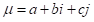(1)

where μ denoted CD, a denoted identity degree and a=S/Nb denoted discrepancy degree and b=F/Nc denoted contradistinction degree and c=P/Ni denoted uncertainty coefficient of discrepancy and the scope of its value was [-1,1], j denoted contradictory coefficient and its value was defined as -1, and it was defined as.

In addition, Eq. 1 showed the calculation of CD of a three-element SPA. However, an SPA can be expanded into a K-element model (Eq. 2),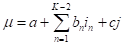(2)

where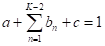, bn denoted different grades of discrepancy degrees, and in denoted a component of the uncertainty coefficient of discrepancy.

In general, the impact of each characteristic was different in the set pair H; therefore, the indices in the SPA of hazard assessment had different weights. The weight of each index can be obtained using AHP (Saaty and Alexander 1989; Guo et al. 2015). The CD considering weight was obtained by Eq. 3,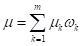(3)

where μ denoted the total CD, ωk denoted the weight of index k, and μk denoted the CD of index k.

Finally, hazard grade was confirmed by the maximal CD principle. For a sample l, assuming the amounts of the corresponding hazard grades were h, its CD was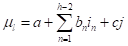. If it was satisfied with the condition d=max{a,b1,b2,…,bh-2,c}, then the hazard grade of sample l can be confirmed as the corresponding grade of d.

GSPA

An SPA can be used for hazard assessment, but it had limits. For example, there was a five-element SPA model, and the corresponding five hazard grades were very low hazard, low hazard, middle hazard, high hazard, and very high hazard, assuming the CD μ1 and μ2 were given as Eq. 4.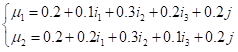(4)

Obviously, the hazard grade of μ1 and μwere both ‘middle hazard’, but the value of μ1 and μ2were definitely different. Hence, the actual hazard grades of μ1 and μ2 were different, but the SPA could not identify the difference. However, if there was an index k, the corresponding hazard grades of it would be very low hazard [0,2], low hazard [2,4], middle hazard [4,6], high hazard [6,8], and very high hazard [8,10]. For a sample l, when its index value was 0.01 or 1.99, the hazard grade of both was ‘very low hazard’. Obviously, the hazard referred to as ‘1.99’ was higher than ‘0.01’ and the SPA could not identify the difference. Consequently, the limitations of the SPA were a lack of precise judgment of the hazard degree and the cursory data handling leading to an incorrect judgment of the hazard grade. Thus, GSPA was proposed to overcome these problems.

At the beginning of GSPA, CMD was introduced. The CMD was used to reflect the connection between the sample and the hazard grade in each index, and its value range was defined as [-1,1] (Li et al. 2011). In this study, the cosine function was introduced to calculate CMD. If the GSPA was using the K-element model, the number of grades was K, and the amounts of thresholds was K+1. Assuming the GSPA was a five-element model, the grades were I, II, III, IV, and V. The corresponding thresholds were set as s0s1s2s3s4, and s5. The calculation algorithm of the CMD is shown below.

1. For sample l and index k, if the index value xkl was bigger when the index k was in higher grade, the calculation algorithm of CMD is below.

Equation 5 was used to calculate the CMD for grade I.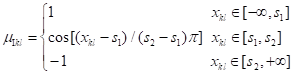(5)

Equation 6 was used to calculate the CMD for grade II.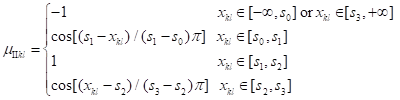(6)

Equation 7 was used to calculate the CMD for grade III.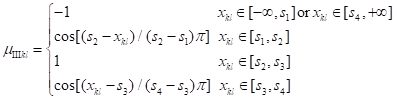(7)

Equation 8 was used to calculate the CMD for grade IV.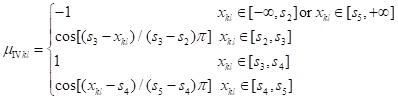(8)

Equation 9 was used to calculate the CMD for grade V.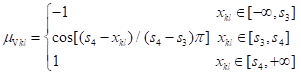(9)

1. For sample l and index k, if the index value xkl was smaller when the index k was in higher grade, the calculation algorithm of the CMD was as shown below.

Equation 10 was used to calculate the CMD for grade I.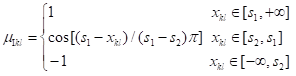(10)

Equation 11 was used to calculate the CMD for grade II.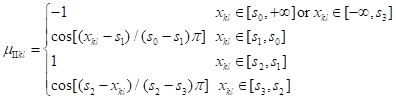(11)

Equation 12 was used to calculate the CMD for grade III.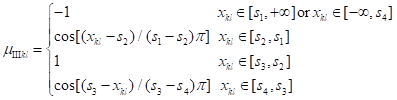(12)

Equation 13 was used to calculate the CMD for grade IV.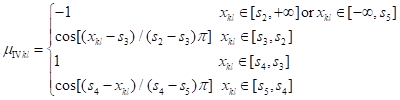(13)

Equation 14 was used to calculate the CMD for grade V.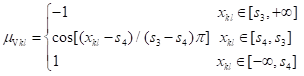(14)

The total CMD of each sample was calculated by Eq. 15.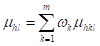(15)

where h denoted the hazard grade, m denoted the amount of index, and ωk denoted the weight of index k.

After the CMD was obtained, an optimization identification model was proposed. As previously mentioned, when the value of μhl was closer to 1, the sample l was closer to grade h. In contrast, if the value of μhl was closer to -1, the sample l was further from grade h. Therefore, deviation degree (DD), WDD, and RMD were introduced to describe the closeness level of sample l to grade h (Eq. 16).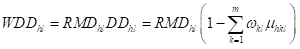(16)

where DDhl denoted the DD of sample l to grade h, WDDhl denoted the WDD of sample l to grade hRMDhl denoted RMD of sample l to grade h and it was regarded as the weight.

To obtain the optimal value of RMDhl, the quadratic sum of the WDD was set as the objective function, with the WDD aimed at the whole samples and each hazard degree (Eq. 17),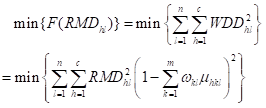(17)

where n denoted the amount of sample and c denoted the amount of hazard grade.

The target was tantamount to solving the minimum value of Eq. 17 under the condition below.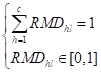(18)

Thus, the Lagrange function was constructed based on the optimization method (Eq. 19),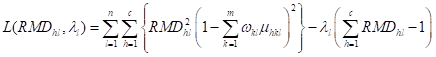(19)

where λl denoted the Lagrange multiplier.

After that, Eq. 19 was solved and the RMD was obtained (Eq. 20).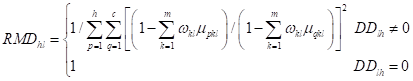(20)

RMD can just reflect the relationship of the sample to each hazard grade; therefore, the CI was introduced to describe the overall characteristics of each sample (Eq. 21),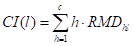(21)

where CI(l) denoted the CI of sample lh denoted the value of hazard grade, for example, when the hazard grade was ‘I’, the value of h was ‘1’, and if the hazard grade was ‘V’, the value of h was ‘5’.

At last, the obtained CI(l) can reveal the numerical hazard of sample l. When the value of CI(l) was bigger, the hazard was higher and when the value of CI(l) was smaller the hazard was lower. For example, assume for two samples l1 and l2, the corresponding values of CI(l) were CI(l1)=2.4 and CI(l2)=2.6. This meant that the hazard grade of the two samples was between grade ‘II’ and grade ‘III’, and the hazard of sample l2 was higher than sample l1.

To sum up, the steps of hazard assessment for biomass gasification stations using GSPA are listed below.

1. Confirmation of assessment indices and the corresponding index weight.
2. Corresponding data collection of biomass gasification stations.
3. Calculate CMD, RMD, and CI.
4. Determination of the hazard grade of biomass gasification stations.

Case Study

In this study, Huangtukan Village biomass gasification station and Yanjia Village biomass gasification station (hereafter referred to as ‘Huangtukan station’ and ‘Yanjia station’; Fig. 1; Fig. 2) were introduced to perform a hazard assessment using GSPA. The two stations were located in Shenyang City, Liaoning Province, Northeast China. Huangtukan station was located at 122.767°E, 41.718°N, and Yanjia station was located at 123.750°E, 41.996°N.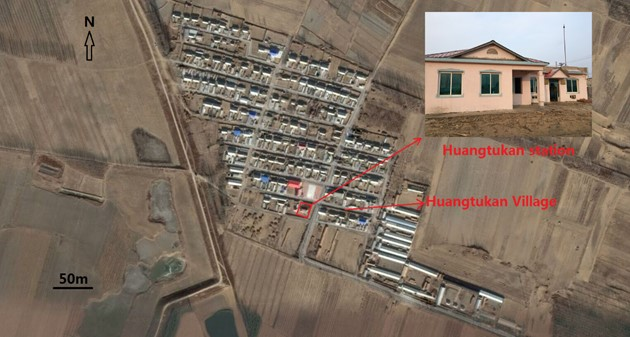Fig. 1. Huangtukan station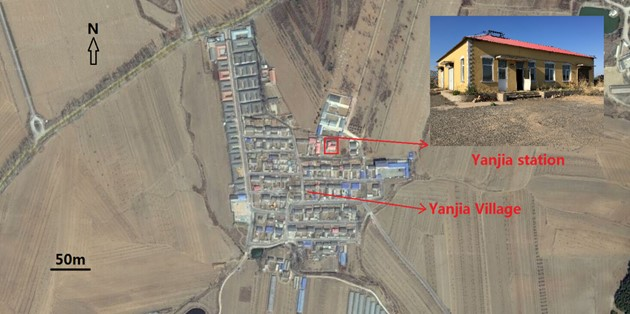Fig. 2. Yanjia station

Confirmation and Classification of Assessment Indices

In the biomass gasification system, biomass materials were used to make biomass gas (biogas). The generated biogas contained the flammable gases hydrogen (H2), carbon monoxide (CO), and methane (CH4) with CO having a high poisonousness as well (Sreejith et al. 2013). Hence, the risks of biomass gasification were fires, explosions, and poisoning. According to the immediate causes of fires, explosions, and poisoning in biomass gasification stations, the 6 corresponding assessment indices (biomass gas production rate (k1), volume fraction of CO (k2), lower explosive limit of biomass gas (k3), artificial ventilation atmosphere (k4), pressure relief ratio (k5) and quantity of biomass materials (k6)) were extracted.

Figure 3 shows the classifications of the assessment indices. For the confirmation of the weight for each index by AHP, let hazard assessment of biomass gasification be the overall objective. Then fires (p1), explosions (p2), and poisoning (p3) were set to be the middle factors. Meanwhile, the 6 corresponding assessment indices were set to be the criteria in AHP (Fig. 3).

After that, the pair-wise comparisons were made and the judgment matrix was then obtained (Guo et al. 2015). The 1/9-9 scale was established to make comparison. That is to say, assuming the two criteria m1 and m2 were set to be compared, if the two criterion were equally important, then the judgment values in the judgment matrix would be. In addition, if the criteria m1 was more important than m2, and the important degree was the highest, then the judgment values in the judgment matrix would beand.

As a result, the judgment matrix for fires, explosions, and poisoning to the overall objective was shown as Eq. 22. The judgment matrices for the 6 corresponding assessment indices to fires, explosions, and poisoning are showed as Eq. 23, Eq. 24, and Eq. 25, respectively. Finally, the weight of each index was confirmed by AHP (Saaty and Alexander 1989; Table 1).

In addition, consistency ratio (CR) of the judgment matrix was calculated based on AHP. CR was used to estimate the judgment matrix. If the value of CR was less than 0.1, then the judgment matrix had satisfactory consistency; otherwise, the judgment matrix has unsatisfactory consistency, and it must be adjusted. In this study, the values of CR of the judgment matrices M1M2M3, and M4 were 0.0176, 0.0039, 0.0462, and 0.0176. Obviously, these judgment matrices had satisfactory consistency.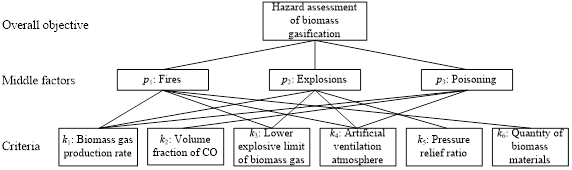Fig. 3. The classifications of the assessment indices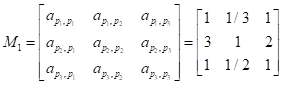(22)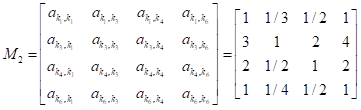(23)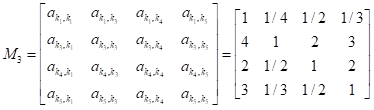(24)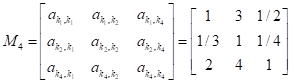(25)

Table 1. Weight of IndexIndex k1 denoted the volume of produced biomass gas per hour (m3/h). Index k2 denoted the volume fraction of CO in the produced biomass gas (%). Index k3 denoted the lower explosive limit of the produced biomass gas (%). Index k4 was reflected by the air change rate (time/h). Index k5 was the ability of pressure relief when the biomass gasification station met fires and explosions, and it was calculated using Eq. 26 (China tMoPSotPsRo 2014),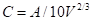(26)

where was the pressure relief ratio (m2/m3), A was the area of pressure relief (m2), and V was the volume of the biomass gasification station (m3). Index k6 was the quantity of biomass materials stored in the biomass gasification station (m3).

In this study, the GSPA was set to be a 5-element model, and the corresponding hazard grades were very low hazard (I), low hazard (II), middle hazard (III), high hazard (IV), and very high hazard (V). The classification of hazard grades is given in Table 2, and the classification was based on the related standard of PRC (China MoAotPsRo 2001; China MoAotPsRo 2005; China tMoPSotPsRo 2014).

Table 2. Classification of Hazard Grade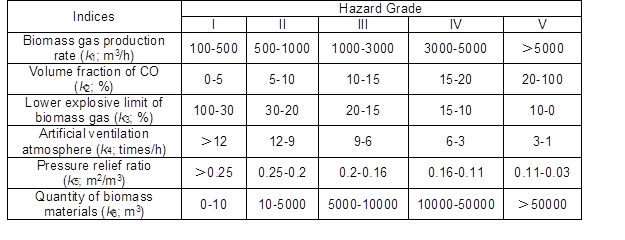Data Collection

The data of Index k1 and k4 were obtained immediately from the construction parameter of the biomass gasification station. The data of Index k2 and k3 were collected by measuring the produced biogas. The data of Index k5 were calculated using the construction and size of the two biomass gasification stations and Eq. 26; where the area of pressure relief was equal to the area of windows and doors (China tMoPSotPsRo 2014). As shown in Figs. 4 and 5, the area of pressure relief for Huangtukan and Yanjia stations was 17.22mand 46.82m2, respectively. The volume of the Huangtukan and Yanjia biomass gasification stations was 149.18 m3 and 315.16 m3, respectively.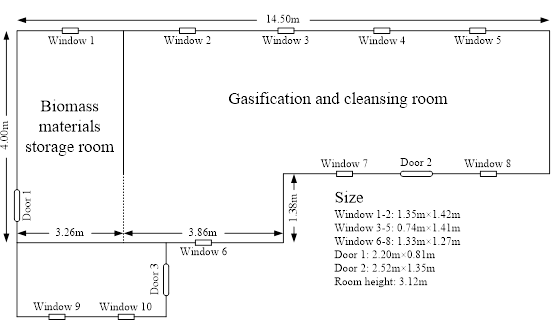Fig. 4. Size of Huangtukan station

As it can be seen in Fig. 4, it needed to be noted that the location of the windows 9-10 and the door 3 was an independent room, and the room was divided off from the biomass materials storage room and the gasification and cleaning room by a wall. Hence, the independent room was left aside in the calculation of pressure relief ratio of Huangtukan station. Then the pressure relief ratio was calculated by Eq. 26. The data of Index k6 were confirmed by the volume of the biomass materials storage room. In general the value of k6 was set as one third of the volume of the biomass materials storage room. All data are listed in Table 3.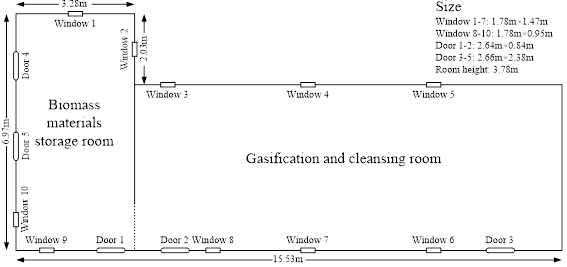Fig. 5. Size of Yanjia station

Table 3. Index Data of Huangtukan station and Yanjia station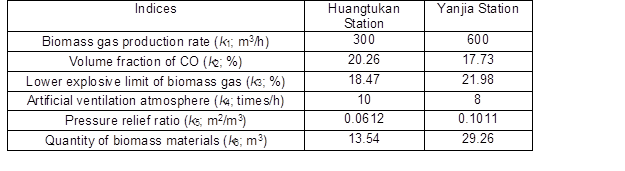RESULTS AND DISCUSSION

Calculation of CMD

The CMD was obtained from Table 3 and Eqs. 5 through 14 (Table 4). The total CMD was computed using Table 1 and Eq. 15 (Table 5).

The assessment results revealed that the hazard grades of Huangtukan station and Yanjia station were both between ‘low hazard’ (grade II) and ‘middle hazard’ (grade III), with the hazard grades falling closer to ‘middle hazard’. The value of the CI also implied that the hazard of Yanjia station was higher than that of Huangtukan station.

Table 4. Calculation of CMD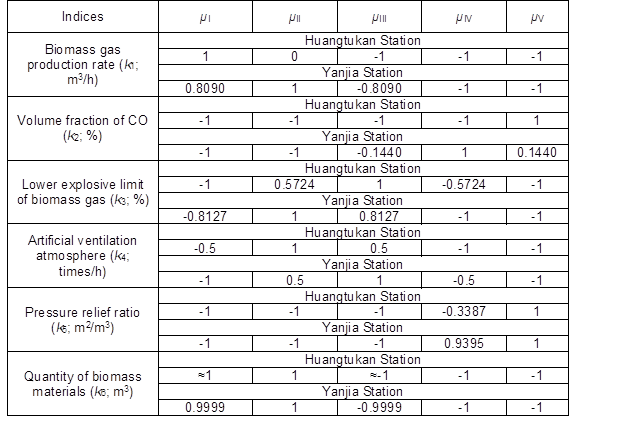Table 5. Calculation Result of Total CMD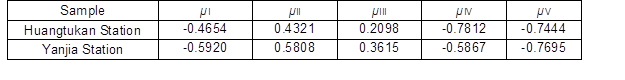Calculation of RMD and CI

After the CMD was obtained, the RMD and CI were obtained using Table 5 and Eqs. 20 through 21 for further analysis (Tables 6 and 7).

Table 6. Calculation of RMD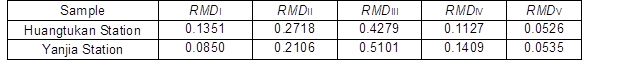Table 7. Calculation of CIComparison of Assessment Results between GSPA and SPA

As an improved hazard assessment method based on SPA, GSPA was proposed in this study. The essence of SPA and GSPA in the hazard assessment was similar, i.e., their aims were both the assessment of the hazard data of the hazard assessment objective and the confirmation of the hazard grade. However, there are some shortcomings of SPA, as previously mentioned, and the proposed GSPA can overcome these shortcomings. In order to discuss the advantage of GSPA over SPA, the comparison of assessment results between GSPA and SPA was made. Then SPA was employed to evaluate the hazard of Huangtukan station and Yanjia station. Equations 2 and 3 and Table 1 were used to calculate the CD based on the maximal CD principle (Table 8).

Table 8. SPA Results of Huangtukan station and Yanjia Station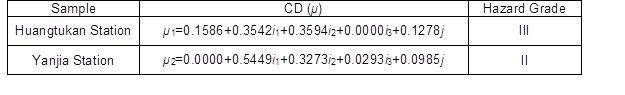The SPA results showed the hazard grade of Huangtukan station was ‘middle hazard’ (grade III), and the hazard grade of Yanjia station was ‘low hazard’ (grade II). The hazard grade of Huangtukan station was higher than that of Yanjia station. As previously mentioned, SPA has shortcomings. For example, for the index k5 (pressure relief ratio), the value of Yanjia station was 0.1011, whereas the SPA just regarded the value as grade V. In actuality the value was very close to the boundary between grades IV and V, and the SPA did not recognize the difference. However, GSPA overcame this problem using CMD calculations. However, for the CD obtained using an SPA, the hazard grade was confirmed only by the maximal coefficient based on the maximal CD principle. For example, for the CD of Huangtukan station, the coefficients of i1 and i2 were almost the same, but the hazard grade was confirmed by the slightly bigger coefficient of i2. These shortcomings made the assessment results incomplete. This is the reason for the differing assessment results between SPA and GSPA. In addition, the assessment results can just reflect the hazard grade given by SPA, while GSPA can make the assessment results more quantitative. For example, the assessment results of Huangtukan station and Yanjia station by GSPA showed that the same hazard grades, but the value of the CI revealed that the hazardousness of Yanjia station was higher.

The above analysis showed that GSPA was an effective and useful method for evaluating the hazardousness of a biomass gasification station. These hazard assessment results can be used as a reference when determining the acceptance inspection standard for the built biomass gasification stations.

Limitations and Prospects

In this study, 6 assessment indices were established to carry out a hazard assessment for biomass gasification stations using GSPA. However, the entire hazard causes of biomass gasification stations can’t just be reflected by the 6 assessment indices. Many causes will lead to fires, explosions, and poisoning in biomass gasification stations, such as the tar, human error, weather factors, etc. As the bottleneck of biomass gasification technology, the tar is especially critical. Whereas the above causes were not the immediate causes of fires, explosions, and poisoning in biomass gasification stations. For example, as one of the ingredients of the produced biomass gas, the tar will lead to the blocking of equipment, and then the blocking may lead to the damage of equipments and gas leakage, and the gas leakage may lead to fires, explosions, and poisoning in biomass gasification stations immediately. To sum up, the tar was an indirect cause of fires, explosions, and poisoning. Similarly, the other causes such as human error and weather factors are also indirect causes of fires, explosions, and poisoning. As previously mentioned, the hazard assessment for the biomass gasification station using GSPA was made according to the immediate causes of fires, explosions, and poisoning in this study, and the hazard assessment can be regarded as a specific hazard assessment based on the immediate causes. For the further work of our study, the indirect causes including the tar, human error, weather factors and so on can be considered to make a more comprehensive hazard assessment for the biomass gasification station.

CONCLUSIONS

1. GSPA is a novel approach based on the study of the use of an SPA, and it was found to be suitable for hazard assessments of biomass gasification stations. The maximal CD principle was abandoned by the GSPA to make hazard assessment. Instead, the GSPA made an overall consideration for each coefficient to obtain the final hazard assessment result. Moreover, the data handling was more accurate by GSPA. Hence, the hazard assessment results found after using the GSPA are much more effective and scientific.
2. The collected data can be handled effectively by the calculation of CMD. It overcame the shortcomings of the SPA in terms of data handling within the same hazard grade and will enhance the accuracy of hazard assessment results. Also, insufficient data utilization can be overcome by the calculation of WDD, RMD, and CI. Thus each coefficient of CMD will be integrated to make a precise hazard assessment result by the calculation of WDD, RMD, and CI.
3. In contrast to SPA, the hazard assessment results of GSPA used numerical values instead of grades to describe the hazardousness of biomass gasification stations. Therefore, it is more convenient to make a comparison of the degree of hazard between two or more biomass gasification stations.

ACKNOWLEDGEMENTS

The study is supported by Rural Energy Comprehensive Construction Foundation of the Ministry of Agriculture of the People’s Republic of China (Grant No. 2015-36).

REFERENCES CITED

Bourenane, H., Guettouche, M. S., Bouhada, Y., and Braham, M. (2016). “Landslide hazard mapping in the Constantine city, Northeast Algeria using frequency ratio, weighting factor, logistic regression, weights of evidence, and analytical hierarchy process methods,” Arabian Journal of Geosciences 9(2), 153-176. DOI: 10.1007/s12517-015-2222-8

Cao, G. L., Zhang, X. Y., Wang, Y. Q., and Zheng, F. C. (2007). “Estimation of emissions from field burning of crop straw in China,” Chinese Science Bulletin 53(5), 784-790. DOI: 10.1007/s11434-008-0145-4

Cherni, J. A., and Kentish, J. (2007). “Renewable energy policy and electricity market reforms in China,” Energy Policy 35, 3616-3629. DOI: 10.1016/j.enpol.2006.12.024

China MoAotPsRo. (2001). “Technical specification and acceptance of standard for straw gasification system of central gas supply (NY/T 443-2001),” In: Ministry of Agriculture of the People’s Republic of China, Beijing

China MoAotPsRo (2005). “Construction criterion for station of argo-residues gasification (NYJ/T 09-2005),” Ministry of Agriculture of the People’s Republic of China, Beijing.

China tMoPSotPsRo (2014). “Code for fire protection design of buildings (GB 50016-2014),” Ministry of Housing and Urban-Rural Development of the People’s Republic of China, Beijing.

Christoforou, E. A., and Fokaides, P. A. (2015). “A review of quantification practices for plant-derived biomass potential,” International Journal of Green Energy 12(4), 368-378. DOI: 10.1080/15435075.2014.880147

Cummer, K. R. and Brown, R. C. (2002). “Ancillary equipment for biomass gasification,” Biomass & Bioenergy 23, 113-128. DOI: 10.1016/S0961-9534(02)00038-7

Du, C. Y., Yu, J. J., Zhong, H. P., and Wang, D. D. (2015). “Operating mechanism and set pair analysis model of a sustainable water resources system,” Frontiers of Environmental Science & Engineering 9(2), 288-297. DOI: 10.1007/s11783-014-0642-4

Gao, X. Y., Zhang, Y. N., Li, B. X., and Yu, X. Y. (2016). “Model development for biomass gasification in an entrained flow gasifier using intrinsic reaction rate submodel,” Energy Conversion and Management 108, 120-131. DOI: 10.1016/j.enconman.2015.10.070

Guo, B. B., Jin, X. B., Yang, X. H., Guan, X., Lin, Y. N., and Zhou, Y. K. (2015). “Determining the effects of land consolidation on the multifunctionality of the cropland production system in China using a SPA-fuzzy assessment model,” European Journal of Agronomy 63, 12-26. DOI: 10.1016/j.eja.2014.11.002

Gupta, J. P., Khemani, G., and Mannan, M. S. (2003). “Calculation of fire and explosion index (F&EI) value for the Dow guide taking credit for the loss control measures,” Journal of Loss Prevention in the Process Industries 16(4), 235-241. DOI: 10.1016/S0950-4230(03)00044-5

Huang, C. F. (1996). “Fuzzy risk assessment of urban natural hazards,” Fuzzy Sets and Systems83(2), 271-282.

Jensen, N., and Jorgensen, S. B. (2007). “Taking credit for loss control measures in the plant with the likely loss fire and explosion index (LL-F&EI),” Process Safety and Environmental Protection 85(B1), 51-58. DOI: 10.1205/psep06001

Jiang, X., Xu, S. G., Liu, Y. Y., and Wang, X. D. (2015). “River ecosystem assessment and application in ecological restorations: A mathematical approach based on evaluating its structure and function,” Ecological Engineering 76, 151-157. DOI: 10.1016/j.ecoleng.2014.04.027

Kazakis, N., Kougias, I., and Patsialis, T. (2015). “Assessment of flood hazard areas at a regional scale using an index-based approach and Analytical Hierarchy Process: Application in Rhodope-Evros region, Greece,” Science of the Total Environment 538, 555-563. DOI: 10.1016/j.scitotenv.2015.08.055

Li, P. Y., Qian, H., and Wu, J. H. (2011). “Application of SPA method based on entropy weight in groundwater quality assessment – A case study in Dongsheng City, Northwest China,” E-Journal of Chemistry 8(2), 851-858. ISSN: 0973-4945

Li, C. H., Sun, L., Jia, J. X., Cai, Y. P., and Wang, X. (2016). “Risk assessment of water pollution sources based on an integrated k-means clustering and set pair analysis method in the region of Shiyan, China,” Science of the Total Environment 557, 307-316. DOI: 10.1016/j.scitotenv.2016.03.069

Lv, X. J., Lu, C. H., Zhu, X. J., and Weng, Y. W. (2015). “Safety analysis of a solid oxide fuel/gas turbine hybrid system fueled with gasified biomass,” Journal of Fuel Cell Science and Technology 12(1), 1-6. DOI: 10.1115/1.4029084

Ogunkoya, D., and Fang, T. G. (2015). “Engine performance, combustion, and emissions study of biomass to liquid fuel in a compression-ignition engine,” Energy Conversion and Management95, 342-351. DOI: 10.1016/j.enconman.2015.02.041

Saaty, T. L., and Alexander, J. M. (1989). “Conflict resolution: The analytic hierarchy process,” New York, Praeger Publishers.

Sreejith, C. C., Muraleedharan, C., and Arun, P. (2013). “Thermo-chemical analysis of biomass gasification by Gibbs free energy minimization model-part: II (optimization of biomass feed and steam to biomass ratio),” International Journal of Green Energy 10(6), 610-639. DOI: 10.1080/15435075.2012.709203

Su, M. R., Yang, Z. F., Chen, B., and Ulgiati, S. (2009). “Urban ecosystem health assessment based on energy and set pair analysis – A comparative study of typical Chinese cities,” Ecological Modelling 220(18), 2341-2348. DOI: 10.1016/j.ecolmodel.2009.06.010

Tao, J., Fu, M. C., Sun, J. J., Zheng, X. Q., Zhang, J. J., and Zhang, D. X. (2014). “Multifunctional assessment and zoning of crop production system based on set pair analysis-A comparative study of 31 provincial regions in mainland China,” Communications in Nonlinear Science and Numerical Simulation 19(5), 1400-1416. DOI: 10.1016/j.cnsns.2013.09.006

Vadrevu, K. P., Eaturu, A., and Badarinath, K. V. S. (2010). “Fire risk evaluation using multicriteria analysis-a case study,” Environmental Monitoring and Assessment 166(1-4), 223-239. DOI: 10.1007/s10661-009-0997-3

Wang, W. S., Jin, J. L., Ding, J., and Li, Y. Q. (2009). “A new approach to water resources system assessment – set pair analysis method,” Science in China Series E-Technological Sciences 52(10), 3017-3023. DOI: 10.1007/s11431-009-0099-z

Wang, W. S., and Li, Y. Q. (2012). “Hazard degree assessment of landslide using SPA method,” Natural Hazards 60(2), 367-379. DOI: 10.1007/s11069-011-0017-0

Wang, M. W., Xu, X. Y., Li, J., Jin, J. L., and Shen, F. Q. (2015). “A novel model of set pair analysis coupled with extenics for evaluation of surrounding rock stability,” Mathematical Problems in Engineering, 1-9. DOI: 10.1155/2015/892549

Wang, T., Chen, J. S., Wang, T., and Wang, S. (2015). “Entropy weight-set pair analysis based on tracer techniques for dam leakage investigation,” Natural Hazards 76(2), 747-767. DOI: 10.1007/s11069-014-1515-7

Wei, C., Dai, X. Y., Ye, S. F., Guo, Z. Y., and Wu, J. P. (2016). “Prediction analysis model of integrated carrying capacity using set pair analysis,” Ocean & Coastal Management 120, 39-48. DOI: 10.1016/j.ocecoaman.2015.11.011

Yue, W. C., Cai, Y. P., Rong, Q. Q., Li, C. H., and Ren, L. J. (2014). “A hybrid life-cycle and fuzzy-set-pair analyses approach for comprehensively evaluating impacts of industrial wastewater under uncertainty,” Journal of Cleaner Production 80, 57-68. DOI: 10.1016/j.jclepro.2014.05.097

Zhang, O., Yu, S. K., and Liu, P. K. (2015). “Development mode for renewable energy power in China: Electricity pool and distributed generation units,” Renewable and Sustainable Energy Reviews 44, 657-668. DOI: 10.1016/j.rser.2015.01.020

Zhao, K. Q. (1994). “Set pair analysis and its preliminary application,” Exploration of Nature, 13(47), 67-72. (in Chinese)

Zhao, K. Q. (2000). “Set pair analysis and its preliminary applications,” Science and Technology Press of Zhejiang, Hangzhou. (in Chinese)

Zou, Q., Zhou, J. Z., Zhou, C., Guo, J., Deng, W. P., Yang, M. Q., and Liao, L. (2012). “Fuzzy risk analysis of flood disasters based on diffused-interior-outer-set model,” Expert Systems with Applications 39(6), 6213-6220. DOI: 10.1016/j.eswa.2011.12.008

Zou, Q., Zhou, J. Z., Zhou, C., Song, L. X., and Guo, J. (2013). “Comprehensive flood risk assessment based on set pair analysis-variable fuzzy sets model and fuzzy AHP,” Stochastic Environmental Research and Risk Assessment 27(2), 525-546. DOI: 10.1007/s00477-012-0598-5

Article submitted: May 24, 2016; Peer review completed: July 18, 2016; Revised version received: August 1, 2016; Accepted: August 4, 2016; Published: August 11, 2016.

DOI: 10.15376/biores.11.4.8307-8324